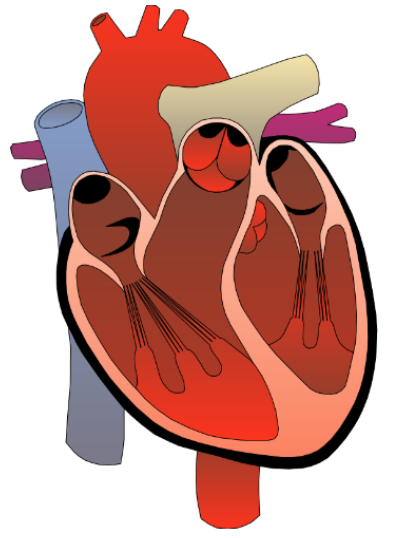# Applications of Calculus: Cardiac OutputCalculus can be used to measure cardiac output, the volume of blood the heart pumps out into the aorta per unit time.

This is done via the dye dilution method, which involves injecting a known volume of dye into the blood, plotting the concentration of the dye as a function of time, and using the concentration function to figure out the blood’s rate of flow.

To figure out the rate of flow, we set up an equation using the following variables:

• $A =$ the initial volume of dye
• $c(t) =$ the dye's concentration as a function of time
• $F =$ the volume of blood pumped out per unit time, i.e. the blood's rate of flow
• $T =$ the amount of time until the dye runs out

The amount of dye pumped out by the heart at any given point in time is calculated as $F \cdot c(t),$ the product of the volume of blood pumped out at that time and the concentration of dye in the blood at that time.

Once the dye runs out, the entire initial volume of dye should have been pumped out. Thus, $A$ should equal the sum of $F \cdot c(t)$ at all points in time, from $0$ to $T.$ We calculate the sum via integration:

\begin{align*} A = \int_0^T F \cdot c(t) dt \end{align*}

Because $F$ is a constant, we can move it out of the integral:

\begin{align*} A = F\int_0^T c(t) dt \end{align*}

Dividing, we reach a formula for $F:$

\begin{align*} F = \frac{A}{\int_0^T c(t) dt} \end{align*}

Tags: